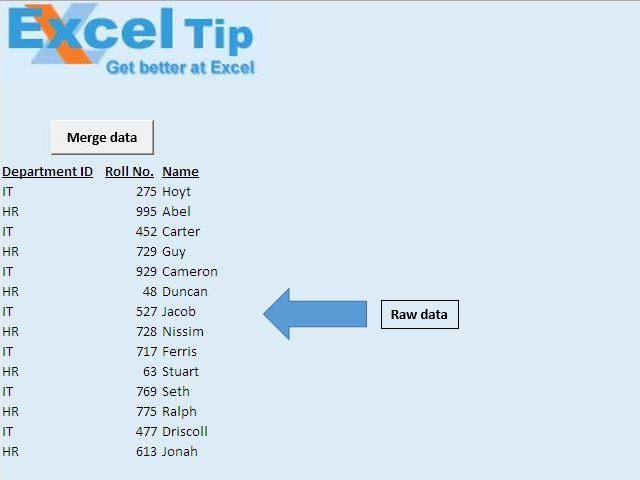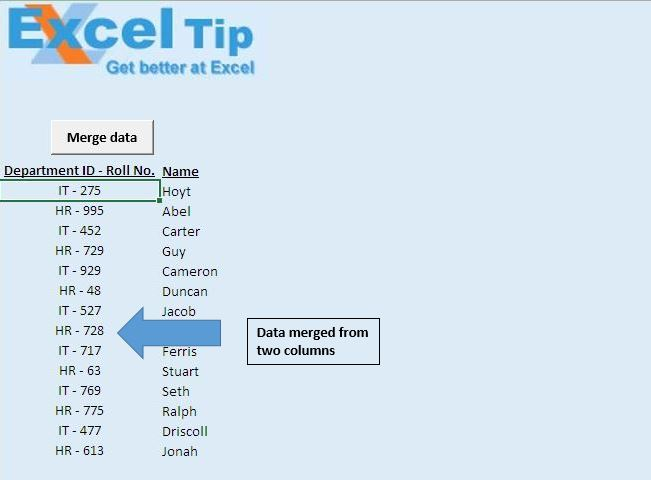# Merge cells without loosing values using VBA in Microsoft Excel

In this article, we will create a macro to merge values in two consecutive cells.

Raw data consists of departmental data, which consists of Department ID, Row Number and Name.In this article, we want to merge Department ID and Roll Number to a single column.Code explanation

Do Until IsEmpty(Cells(IntRow, IntCol))

Loop

The above code is used to loop until an empty cell is found.

Cells(IntRow, IntCol) = Cells(IntRow, IntCol) & " - " & Cells(IntRow, IntCol + 1)

The above code is used to concatinate values into a single cell, separated by “-”.

Cells(IntRow, IntCol + 1).ClearContents

The above code is used to delete the content from the cell.

Range(Cells(IntRow, IntCol), Cells(IntRow, IntCol + 1)).Merge

The above code is used to merge two consecutive cells together.

With Selection

.HorizontalAlignment = xlCenter

.VerticalAlignment = xlCenter

End With

The above code is used to center allign the text horizontally and verticaly.

```
Option Explicit

Sub Connects()

'Declaring variables
Dim IntRow, IntCol As Integer

'Initializing row and column number of first cell
IntRow = 9
IntCol = 1

Application.ScreenUpdating = False

'Looping through cells until blank cell is encountered in first column
Do Until IsEmpty(Cells(IntRow, IntCol))
'Merging value from two cells in the first column
Cells(IntRow, IntCol) = Cells(IntRow, IntCol) & " - " & Cells(IntRow, IntCol + 1)
'Clearing content from second column
Cells(IntRow, IntCol + 1).ClearContents
'Merging two cells
Range(Cells(IntRow, IntCol), Cells(IntRow, IntCol + 1)).Merge
'Moving to next row
IntRow = IntRow + 1
Loop

'Formatting the first column
Columns(IntCol).Select
'Setting the horizonatal and vertical alignment to center
With Selection
.HorizontalAlignment = xlCenter
.VerticalAlignment = xlCenter
End With

Range("A10").Select

End Sub

```

We would love to hear from you, do let us know how we can improve our work and make it better for you. Write to us at info@exceltip.com

1.I agree. It would be complete to enable the macro to run on a selected range. The changes could include replacing the "-" sign between the contents of the cells with a space. (This is can be done by almost anyone).

2."This just saved me a ton of time, thank you so much for posting!! If you ever edit this, it might be nice to enable the macro to either run on a certain range or an entire worksheet.

Thanks again,
David"

Terms and Conditions of use

The applications/code on this site are distributed as is and without warranties or liability. In no event shall the owner of the copyrights, or the authors of the applications/code be liable for any loss of profit, any problems or any damage resulting from the use or evaluation of the applications/code.